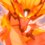# Monster Limit 3

Suppose,

$$\displaystyle f(x) = \sum_{n=0}^{\infty} f_n x^n$$, $$\displaystyle F(n) = \sum_{r=0}^{n} f_{r}$$ and $$\displaystyle \lim_{n \to \infty} \dfrac{F(n)}{n^{r_f}} = \text{d}_f$$

Similarly,

$\displaystyle g(x) = \sum_{n=0}^{\infty} g_n x^n$, $\displaystyle G(n) = \sum_{r=0}^n g_r$ and $\displaystyle \lim_{n \to \infty} \dfrac{G(n)}{n^{r_g}} = \text{d}_g$

From Monster Limit 2, we have,

$\begin{cases} \lim_{x \to 1^{-}} (1-x)^{r_f} \ f(x) = \text{d}_f \ \Gamma(1+r_f)\\ \lim_{x \to 1^{-}} (1-x)^{r_g} \ g(x) = \text{d}_g \ \Gamma(1+r_g) \end{cases}$

Now, consider the same quantities for $fg(x) = f(x)g(x)$, i.e,

$\displaystyle fg(x) = \sum_{n=0}^{\infty} {fg}_n x^n$, $\displaystyle FG(n) = \sum_{r=0}^{n} {fg}_{r}$ and $\displaystyle \lim_{n \to \infty} \dfrac{FG(n)}{n^{r_{fg}}} = \text{d}_{fg}$

Again,

$\displaystyle \lim_{x \to 1^{-}} (1-x)^{r_{fg}} \ fg(x) = \text{d}_{fg} \ \Gamma(1+r_{fg})$

Prove That
$\displaystyle \text{d}_{fg} = \text{d}_{f} \text{d}_{g} \dfrac{\Gamma(1+r_f) \Gamma(1+r_g)}{\Gamma(1+r_f + r_g)}$Note by Ishan Singh
2 years, 9 months ago

This discussion board is a place to discuss our Daily Challenges and the math and science related to those challenges. Explanations are more than just a solution — they should explain the steps and thinking strategies that you used to obtain the solution. Comments should further the discussion of math and science.

When posting on Brilliant:

• Use the emojis to react to an explanation, whether you're congratulating a job well done , or just really confused .
• Ask specific questions about the challenge or the steps in somebody's explanation. Well-posed questions can add a lot to the discussion, but posting "I don't understand!" doesn't help anyone.
• Try to contribute something new to the discussion, whether it is an extension, generalization or other idea related to the challenge.

MarkdownAppears as
*italics* or _italics_ italics
**bold** or __bold__ bold
- bulleted- list
• bulleted
• list
1. numbered2. list
1. numbered
2. list
Note: you must add a full line of space before and after lists for them to show up correctly
paragraph 1paragraph 2

paragraph 1

paragraph 2

[example link](https://brilliant.org)example link
> This is a quote
This is a quote
    # I indented these lines
# 4 spaces, and now they show
# up as a code block.

print "hello world"
# I indented these lines
# 4 spaces, and now they show
# up as a code block.

print "hello world"
MathAppears as
Remember to wrap math in $$ ... $$ or $ ... $ to ensure proper formatting.
2 \times 3 $2 \times 3$
2^{34} $2^{34}$
a_{i-1} $a_{i-1}$
\frac{2}{3} $\frac{2}{3}$
\sqrt{2} $\sqrt{2}$
\sum_{i=1}^3 $\sum_{i=1}^3$
\sin \theta $\sin \theta$
\boxed{123} $\boxed{123}$